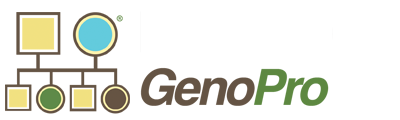GenoPro Home  |  SDK Home  |  Report Generator  |  Support  |  Search  |  Help  |  Site MapConfigurationDictionaryPhraseDocumentGenoCollectionGenoDateGenoObjectGenoRectIndividualLanguageDictionaryNameDictionaryObjectRepertoryReportReportGeneratorSessionStringDictionaryTimeSpanUtilPlugin DemoExternal StorageCoding style

# GenoRect Class

The `GenoRect` class encapsulates a rectangle.

 Property Description `Left` Get or set the left value of the rectangle. `Right` Get or set the right value of the rectangle. `Top` Get or set the top value of the rectangle. `Bottom` Get or set the bottom value of the rectangle. `ToString` Returns a string representing the rectangle. The string returned includes two points in the format "x1, y1 x2, y2". Example: `123,456 678,999` `FormatXY1XY2` Similar to the `ToString` property, however returns a string ready for HTML/XML/SVG attributes. Optionally, you may pass a parameter to inflate the rectangle. Example: `x1="123" y1="456" x2="789" y2="999"` `FormatXYWH` Very similar to `FormatXY1XY2`, however returns a width and height instead of x2, y2. Example: `x1="123" y1="456" width="666" height="543"`

Usage:
Here is an example to use the GenoRect object.

``````
Set rc = i.Position.boundary_rect
Report.WriteBr rc ' Write the default value (implicitly calling ToString)
Report.WriteBr rc.FormatXY1XY2
Report.WriteBr rc.FormatXY1XY2(-10) ' Shrink the rectangle by 10 units on each side

rc.Left = rc.Left + 1 ' Move the left edge by one unit
Report.WriteBr rc.FormatXYWH
Report.WriteBr rc.FormatXYWH(+5) ' Inflate the rectangle by 5 units on each side
``````

Future Improvements:

The methods `FormatXY1XY2` and `FormatXYWH` could have an extra parameter `strFormatOptions` to perform additional formatting:

 strFormatOption Meaning `"` Put double quotes around the values. This is the default value anyways Example: `x1="123" y1="456" x2="789" y2="999"` `'` Put double quotes around the values. Example: `x1='123' y1='456' x2='789' y2='999'` `=` Don't put any quotes around the values. Example: `x1=123 y1=456 x2=789 y2=999` `-` Negate the y-values (Top and Bottom) Example: `x1="123" y1="-456" x2="789" y2="-999"` `, (comma)` Separate the values with a comma instead of a space. Example: `x1="123",y1="456",x2="789",y2="999"`

Of course, you could make combinations such as

`rc.FormatXYWH 10, "-="`

This would return a rectangle inflated by 10 units on each site, with the top and bottom negated and no quotes around the values.